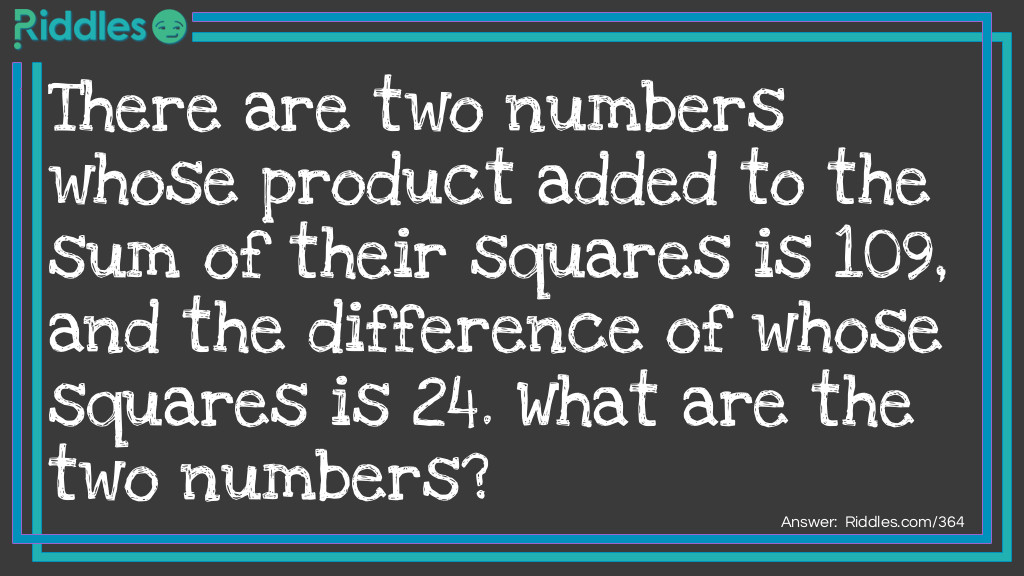# There Are Two Numbers Whose Product Added To The Sum Of Their Squares Is 109...

Author: Anonymous
8 years ago

Riddle: There are two numbers whose product added to the sum of their squares is 109, and the difference of whose squares is 24. What are the two numbers?
Answer: 5 and 7. (5)² = 25(7)² = 49(5x7)+25+49=10949-25=24
VOTE
Source: https://www.riddles.com/364
COMMENTThere are two numbers whose product added to the sum of their squares is 109... by Anonymous v1.